# 紫罗兰光

Violetshine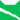雷族 (Q627)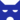影族 (Q628)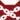至亲 (Q651)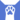反抗者 (Q4414)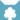天族 学徒天族 武士天族 猫后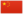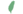小紫罗兰 Violetkit  小紫羅蘭
 紫罗兰爪 Violetpaw  紫羅蘭掌
 紫罗兰光 Violetshine  紫羅蘭光

Q3513：天族母猫，在影族长大，自《学徒探索》登场的角色

## 细节

### 外貌

• 她是一只身形娇小、:14皮毛光泽、:279黄色眼睛、:猫物表黑色斑块的白色:197短毛母猫，有着宽大的脚掌。:197

### 勘误

• 她曾被错误地描述为琥珀色眼睛。:31
• 在晋升为学徒前，她曾被错误地称呼为“紫罗兰爪”。:157

## 登场书目

• 学徒探索 (Q170)[配角][首次登场🐱]
• 雷影交加 (Q171)[主人公][主要角色][视角人物]
• 天空破碎 (Q172)[视角人物]
• 极夜无光 (Q173)[主人公][主要角色][视角人物]
• 烈焰焚河 (Q174)[主人公][主要角色][视角人物]
• 风暴来袭 (Q175)[主人公][主要角色][视角人物]
• 迷失群星 (Q3563)[配角]
• 静默冰融 (Q3579)[配角]
• 叠影重障 (Q3624)[配角]
• 暗由心生 (Q4183)[配角]
• 无星之地 (Q4385)[对话提及]
• 迷雾明光 (Q4621)[配角]
• 河翻浪涌 (Q4643)[对话提及]
• 天穹将倾 (Q4799)[猫物闲角]
• 虎心的阴影 (Q185)[对话提及]
• 松鼠飞的希冀 (Q3561)[配角]
• 灰条的誓言 (Q4057)[猫物闲角]
• 一星的告解 (Q4826)[配角]
• 褐皮的族群 (Q3566)[猫物闲角]
• 卵石光的幼崽 (Q3626)[配角]
• 黛西的至亲 (Q3629)[对话提及]
• 斑毛的反抗 (Q4337)[对话提及]
• ## 外部链接

### 官方文章

• 角色聚光灯－阿树 （提及）
• 一路走来－《风暴来袭》 （提及）
• 《迷失群星》诞生记 （提及）
• 哪名武士最值得？ （隐射）
• 欢迎来到第五族群 （隐射）
• 至尊反派 （提及）
• 创造反派 （隐射）
• 共同的纽带 （提及）
• 你至亲的至亲 （提及）
• 解读征兆 （隐射）
• 写个好预言 （提及）
• 只是故事而已 （隐射）
• 比憎恨更强大 （提及）
• 谨言慎行…… （隐射）
• 真正的武士？ （提及）
• 假如……？ （提及）
• 河族的回归 （提及）
• 致敬鬃霜 （隐射）
• 我们所铭记的死亡 （提及）
• 一路走来－《迷雾明光》 （隐射）
• 平衡光明的黑暗 （提及）
• The Forest Map: WindClan （隐射）
• 换族者：他们会留下吗？ （提及）
• ## 参考文献

这篇文章基于CC BY-SA 3.0许可使用了猫武士维基（英语）Violetshine一文中的部分内容。